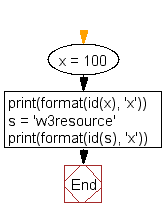﻿ Python: Get variable unique identification number or string - w3resource# Python: Get variable unique identification number or string

## Python List: Exercise - 36 with Solution

Write a Python program to get variable unique identification number or string.

Sample Solution:-

Python Code:

``````x = 100
print(format(id(x), 'x'))
s = 'w3resource'
print(format(id(s), 'x'))
```
```

Sample Output:

```a6aa40
7f209e6c6670
```

Flowchart:## Visualize Python code execution:

The following tool visualize what the computer is doing step-by-step as it executes the said program:

Python Code Editor:

Have another way to solve this solution? Contribute your code (and comments) through Disqus.

What is the difficulty level of this exercise?

Test your Python skills with w3resource's quiz

﻿

## Python: Tips of the Day

Floor Division:

When we speak of division we normally mean (/) float division operator, this will give a precise result in float format with decimals.

For a rounded integer result there is (//) floor division operator in Python. Floor division will only give integer results that are round numbers.

```print(1000 // 300)
print(1000 / 300)```

Output:

```3
3.3333333333333335```